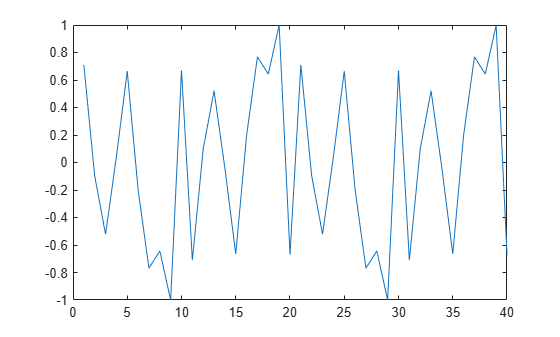# resample

Resample time-domain data by decimation or interpolation (requires Signal Processing Toolbox software)

## Syntax

```resample(data,P,Q) resample(data,P,Q,order) ```

## Description

`resample(data,P,Q)` resamples data such that the data is interpolated by a factor `P` and then decimated by a factor `Q`. `resample(z,1,Q)` results in decimation by a factor `Q`.

`resample(data,P,Q,order)` filters the data by applying a filter of specified `order` before interpolation and decimation.

## Input Arguments

`data`

Name of time-domain `iddata` object. Can be input-output or time-series data.

Data must be sampled at equal time intervals.

`P`, `Q`

Integers that specify the resampling factor, such that the new sample time is `Q/P` times the original one.

`(Q/P)>1` results in decimation and `(Q/P)<1` results in interpolation.

`order`

Order of the filters applied before interpolation and decimation.

Default: `10`

## Examples

collapse all

Increase the sampling rate of data by a factor of 1.5 and compare the resampled and the original data signals.

```u = idinput([20 1 2],'sine',[],[],[5 10 1]); u = iddata([],u,1); plot(u) ur = resample(u,3,2); plot(u,ur) ```## Algorithms

If you have installed the Signal Processing Toolbox™ software, `resample` calls the Signal Processing Toolbox `resample` function. The algorithm takes into account the intersample characteristics of the input signal, as described by `data.InterSample`.